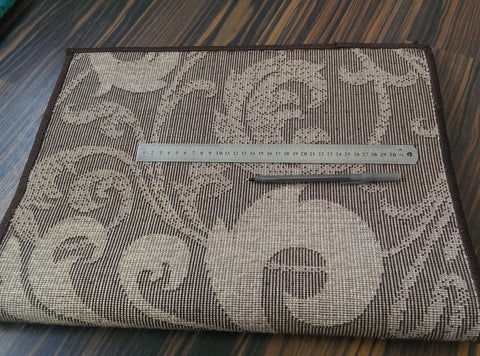# How to Count the Point or Knot Density of a Machine Made Rug

Rug Density and What it Means to You

Rug weaving starts with a weft, or net of yarn. Once this net is set on the loom another yarn, called the warp, is woven through that net. The weft and warp are knotted at each point they intersect, forming the rug. Rug density is a measure of the number of knots used to make a rug.

One way to think about area rug point count is like resolution on a computer screen. The more pixels a screen has the more detail can be displayed. If we think of points of yarn like pixels higher point density means more detailed designs. With this density the weight of the rug increases because more material is used to make the rug.

This count is measured in points per square meter with each point being a single knot. This number is calculated by multiplying the number of knots running horizontally on the weft and vertically on the warp. You can even calculate this yourself.

It’s easy!

All you’ll need is:

• A metric ruler with centimeter markings
• A pencil, pen, or other object with a fine tip to count the individual points.How to do it:

Take your ruler and count the points running 10 centimeters across and 10 down on the back of your rug. Make sure you start from the same point for both measurements.

Take these two numbers and multiply them. Then take this number and multiply it by 100 to get the total number of points in one square meter.

Imagine a rug has 32 points on the weft and 47 on the warp. The calculation would go like this:

(32(weft count) x 47 (warp count) x 2) x 100 = 300,800

So this rug has 300,800 points per square meter.